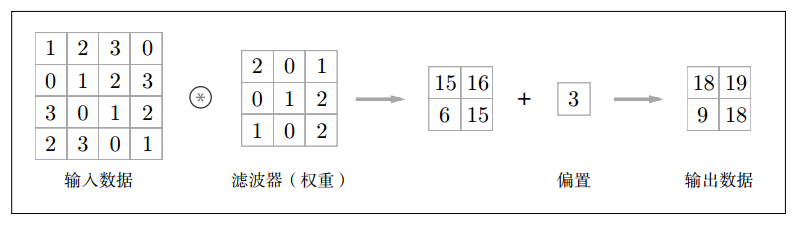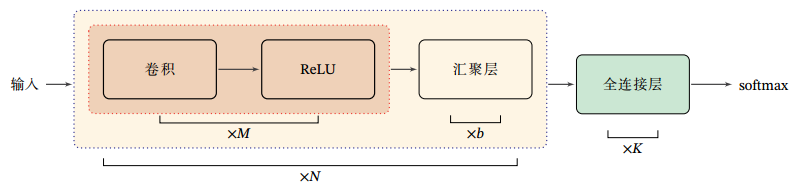# CNN

## 1. 简介

CNN 是专门用于处理网格化数据的神经网络。CNN 中新增了 Convolution 层和 Pooling 层，CNN 的层的连接顺序是「Convolution-ReLU-(Pooling)」（Pooling层有时会被省略）。这可以理解为之前的“Affine-ReLU”连接被替换成了「Convolution-ReLU-(Pooling)」连接。

## 2. CNN 的优势

• 全连接层（Affine 层）忽略了数据的形状。比如，输入数据是图像时，图像通常是高、长、通道方向上的 3 维形状。但是，向全连接层输入时，需要将 3 维数据拉平为 1 维数据。

• 而卷积层可以保持形状不变。当输入数据是图像时，卷积层会以3维数据的形式接收输入数据，并同样以3维数据的形式输出至下一层。因此，在CNN中，可以（有可能）正确理解图像等具有形状的数据。

【注】把 3 维数据表示为多维数组时，书写顺序为（channel, height, width）。作为 4 维数据，滤波器的权重数据要按（output_channel, input_channel, height, width）的顺序书写。

## 3. 术语

• 特征图（feature map）：卷积层的输入输出数据。输入数据称为输入特征图，输出数据称为输出特征图。• 卷积（convolution）：滤波器上的元素和输入的窗口数据对应元素进行相乘并累加求和。

• 填充（padding）：在进行卷积层的处理之前，有时要向输入数据的周围填入固定的数据（比如 0 等）。「幅度为1的填充」是指用幅度为 1 像素的 0 填充周围。

• 步幅（stride）：应用滤波器的位置间隔称为步幅。

【注】增大步幅后，输出大小会变小。而增大填充后，输出大小会变大。

$\begin{array}{c} OH = \frac{H + 2P - FH}{S} + 1 \\ OW = \frac{W + 2P - FW}{S} + 1 \end{array}$

• 池化（pooling）：（也称为汇聚）池化是缩小高、长方向上的空间的运算。一般来说，池化的窗口大小会和步幅设定成相同的值。池化是指对每个区域进行下采样（Down Sampling）得到一个值，作为这个区域的概括。

1. Max 池化
2. Average 池化

## 4. 卷积层

### 4.1 一维卷积

$\begin{array}{c} y_t = \sum_{k=1}^K w_k x_{t-k+1} \end{array}$

$\begin{array}{c} \boldsymbol{y} = \boldsymbol{w} * \boldsymbol{x} \end{array}$

### 4.2 二维卷积

$\begin{array}{c} y_{ij} = \sum_{u=1}^U \sum_{v=1}^V w_{uv} x_{i-u+1,j-v+1} \end{array}$

$\begin{array}{c} \boldsymbol{Y} = \boldsymbol{W} * \boldsymbol{X} \end{array}$

### 4.3 互相关

$\begin{array}{c} y_{ij} = \sum_{u=1}^U \sum_{v=1}^V w_{uv} x_{i+u-1,j+v-1} \end{array}$

$\begin{array}{c} \boldsymbol{Y} = \boldsymbol{W} \otimes \boldsymbol{X} = rotate_{180^\circ}(\boldsymbol{W}) * \boldsymbol{X} \end{array}$

• 在神经网络中使用卷积是为了进行特征抽取，卷积核是否进行翻转和其特征抽取的能力无关。

• 特别是当卷积核是可学习的参数时，卷积和互相关在能力上是等价的。

• 在具体实现上，一般会以互相关操作来代替卷积，从而会减少一些不必要的操作或开销。

### 4.4 常用卷积

• 窄卷积：$S = 1, P = 0$，卷积后输出长度为 $M - K + 1$

• 宽卷积：$S = 1, P = K - 1$，卷积后输出长度为 $M + K - 1$。宽卷积运算符号为：$\tilde{\otimes}$

• 等宽卷积：$S = 1, P = (K-1)/2$，卷积后输出长度为 $M$

### 4.5 导数

$\begin{array}{c} \frac{\partial f(\boldsymbol{Y})}{\partial \boldsymbol{W}} = \frac{\partial f(\boldsymbol{Y})}{\partial \boldsymbol{Y}} \otimes \boldsymbol{X} \\ \frac{\partial f(\boldsymbol{Y})}{\partial \boldsymbol{X}} = rotate_{180^\circ}(W) \tilde{\otimes} \frac{\partial f(\boldsymbol{Y})}{\partial \boldsymbol{Y}} \end{array}$

## 5. 反卷积（转置卷积）层

### 5.1 转置关系

$\begin{array}{c} \boldsymbol{z} = \boldsymbol{W} \boldsymbol{x} \end{array}$

$\begin{array}{c} \boldsymbol{x} = \boldsymbol{W}^T \boldsymbol{z} \end{array}$

【注】在全连接网络中，忽略激活函数，前向计算和反向传播就是一种转置关系。

• 前向计算：第 $l + 1$ 层净输入：

$\begin{array}{c} \boldsymbol{z}^{(l+1)} = \boldsymbol{W}^{(l+1)} \boldsymbol{z}^{(l)} \end{array}$

• 反向传播：第 $l$ 层的误差项：

$\begin{array}{c} \delta^{(l)} = (\boldsymbol{W}^{(l+1)})^T \delta^{(l+1)} \end{array}$

### 5.2 反卷积（转置卷积）

$\begin{array}{c} H_{out}=(H_{in}-1)stride-2padding+kernel\_size+output\_padding \end{array}$

$\begin{array}{c} W_{out}=(W_{in}-1)stride-2padding+kernel\_size+output\_padding \end{array}$

## 6. 池化层

• 没有要学习的参数
• 通道数不发生变化
• 对微小的位置变化具有鲁棒性（健壮）